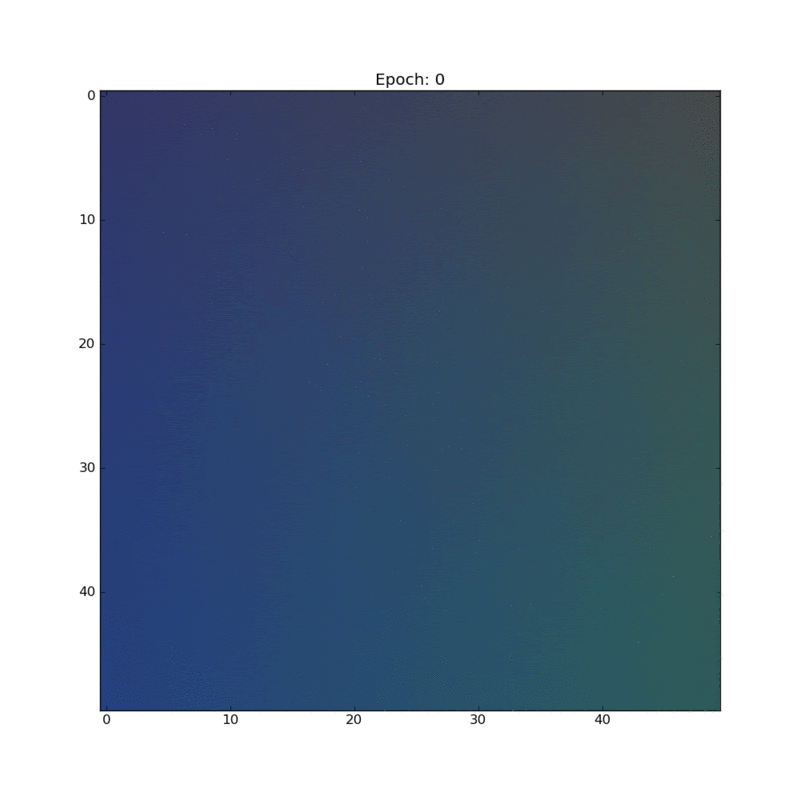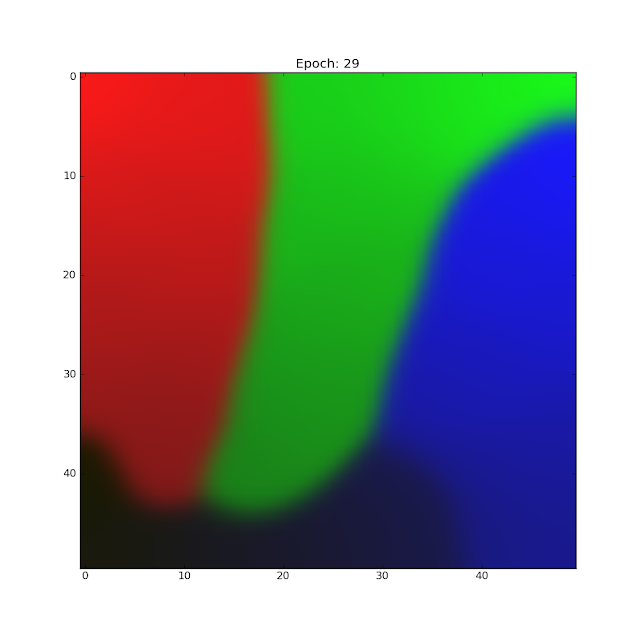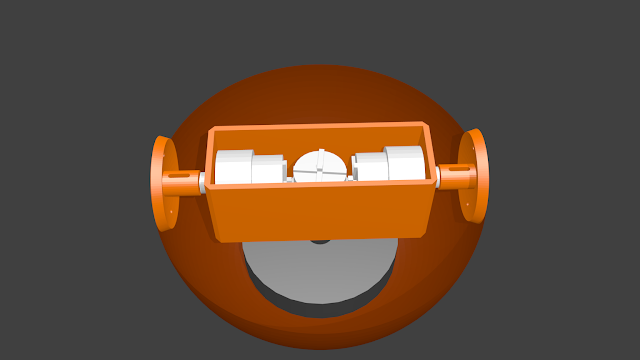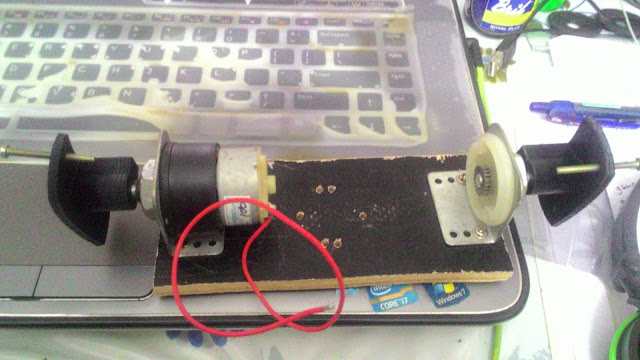## Saturday, 3 December 2016

### Getting Started With STM32 and Simulink

The STM32 microcontrollers are powerful and full of awesome features but as anyone who has tried to work with the STM32 microcontrollers will know, setting them up and programming them can be a little difficult. I recently bought myself the STM32F4 Discovery development board and after trying a lot of new things, managed to setup a working development environment. I initially set up my development environment in Ubuntu. I found that the best option on linux is to use the System Workbench. But I found that getting anything more than a basic LED blink working was quite difficult. You have to pore over the ARM programmers manual and the StdPeripheral reference for hours and hours to get anything at all done. And if your focus is on the development of algorithms, maybe you don't want to spend so much time with the nitty gritty details of the implementation.

Since I joined a PhD program a few months back, I have acess to Matlab from my university. So I decided to see if it was possible to use Simulink (which has support for quite a lot of hardware) would suit my workflow. I first tried the STM32 target for Simulink that's provided by ST themselves. After struggling with it for a week I gave up. I ran into multiple issues. First of all, the library was finnicky and not very easy to use. The user interface was set up in a confusing way. Second, I felt that the library was not complete because it did not implement a lot of features that were available on the processor like the option to read an incremental encoder using the timer. Of course, I guess it's possible to do it if you configure the timer and the pins yourself, but that defeats the purpose of having a Simulink block library in my opinion. Also, some of the blocks kept throwing errors and I was unable to run the examples because they were made for Matlab 2015b and I only had access to 2015a from my university.

Instead I ended up using the waijung blockset for STM32. I've been trying it out for the last few hours and I think it's far better than the official library from ST. It implements most of the features on the STM32 (including incremental encoders) and the setup is a lot more intuitive. I managed to get an LED blinker setup in under an hour.

## Thursday, 16 June 2016

### New Website and Blog

As I mentioned in an earlier post (exactly a year ago! :D) I have my own website now. When I first bought the domain name and hosting package I opted to get a shared hosting server where I could build my own website using Wordpress. However, it never really satisfied me. I got a decent looking website up and running, but I had to work with the constraints of the Wordpress CMS. Whenever I wanted a part of my website to look a particular way, I had to go deep into menus and settings pages to try and tweak it to my liking. Also, most of the themes that were available didn't meet all of my requirements. Some of them would almost meet them but would be missing one critical feature or the other. In the end, I had to compromise by picking a theme that was good enough. I let my website be for about a year as I didn't have time in the middle of the semester to tweak everything to my liking.

In May I finally finished my bachelor's degree. That left me with more time on my hands to tweak my website to my liking. I tried to keep the wordpress blog but I found working with Wordpress themes quite difficult if I wanted to modify them. In the end, I decided to go for something simple that worked: Bootstrap. It was a barebones solution that produced good results using simple HTML classes. I found myself a good theme from Startbootstrap and got started redesigning my website.

Since I was using a static site generator, I no longer needed a hosting service which allowed disk access. So I decided that Github Pages would be the new home of my website. I canceled the hosting service but kept my domain name and pointed it to my brand new github pages site.

I also discovered that it was possible to use Jekyll to produce static blogs that did not require databases. They also had support for importing blogger posts. Jekyll has a lot of cool in built markdown formatting features that Blogger lacks. So I decided to give it a shot. Although I got most of my important posts imported and running on a new Jekyll blog, I was a bit reluctant to leave the platform that I'd been using for so many years. In the end I decided to maintain both blogs separately. The new Jekyll blog will mirror this blog for the most part. However, the Jekyll blog will be curated a little bit more strictly to contain only "professional" posts. This blog will continue to be my outlet for both professional/technical and fun random posts.

Go to ashwinnarayan.com to check out the new website.New BlogNew Website

## Wednesday, 30 March 2016

### Unsupervised Learning: Self Organizing Maps

Most machine learning techniques like logistic regression, linear regression, and neural networks do something that is called supervised learning. This means that we need to supply examples of "correct" data to the machine learning algorithm for it to learn the patterns in the data. The goal of unsupervised learning is to automatically find clusters of similar data in a huge unlabeled set of data. Humans can do this easily with some types of data. If you saw a 2D scatter plot of points you'd easily be able to identify clusters in the data by visual examination. I've always considered unsupervised learning cooler than supervised mostly because it feels like that's what a general AI should be able to do.

Self organizing maps (SOMs) are one technique used to find these clusters in the data. A self organizing map looks a little similar to a neural network at first. But the kind of operation that it does is very different. An SOM has a lattice of nodes. This lattice is usually one dimensional(arranged in a linear array) or 2 dimensional (arranged in a matrix). As the self organizing map is trained, the lattice of will be partitioned into separate classes. Since one of the main uses of self organizing maps is visualizing higher dimensional data, the lattice is is rarely more than two dimensions wide. The clusters in the higher dimensional data can be visualized on the 2D lattice.  Associated with each of the nodes in the lattice is a weight vector that has the same dimension as the input data vectors. To train the self organizing map we take each element in the data set and find the node with the weight that's closest to the element (Using the euclidean distance as the measure of closeness. Other measures can be used too.). Then we define a neighbourhood of nodes that are 'close' to this best matching unit and we adjust the weights of this entire neighbourhood of weights to be a little closer to the value of the element from the dataset. How much the weights are adjusted depends on the neighbour falloff function and on the learning rate. This can be expressed in one simple equation.
$$W_{uv}(t+1) = W_{uv}(t) + \Omega (u,v,t) \alpha (t)[x_n - W_{uv}(t)]$$
In each iteration, the learning rate and the size of the neighbourhood function is reduced by a small amount. The neighbourhood size is initially set to a large value. This allows the algorithm to initially affect the weights on a global scale and then over time adjust smaller and smaller regions of the map to train it.

In my implementation I used a Gaussian neighbourhood function whose variance decayed exponentially. The learning rate started off at 0.1 and decayed exponentially from there.

To check my implementation, I tested something that is a common use for SOMs. Clustering colors in an RGB color space. Colors are easy to visualize and I thought it would make a good demo. I first generated a random data set of bluish, reddish, greenish and dark bluish colors.A random sampling of 4 different colors for the dataset.
I initialized the weights randomly and started the training. For this particular experiment I used a 2D 50x50 lattice of nodes. Since each node has a weight that is a 3 dimensional vector that represents a color, we can visualize the SOM node lattice as an RGB image. Stacking together the weight visualization after each epoch (One pass through the training set) of training gave me this really beautiful looking animation! :DAnimation that shows the self organizing map after each epoch of training.
You can clearly see the different clusters of color slowly resolving as the training happens. The initial changes are more global due to the large neighbourhood. But as the training progresses the adjustments to the weights become more and more local. There is also a very nice separation of the different colors that were present in the first image. You can see that the brighter colors seem to be congregating towards the top of the image and the darker colors towards the bottom. The darkest blue (maybe even a little black) ended up in the bottom left corner surrounded by the darker hues of red, green and blue.The final map after 30 epochs of training.

## Tuesday, 22 March 2016

### Machine Learning Part 2: Implementing Multi Class Logistic Regression

In my last post on machine learning I talked about how to implement simple linear regression in Python. This time I am going to implement logistic regression. This technique is used to classify input data into one of several classes.

First let's take a look at two class regression.

Let's have a set of input vectors $\{x_1, x_2, ... , x_N\}$ and a set of targets $\{t_1, t_2, t_3, ..., t_N\}$ as our training data. A logistic regression classifier defines a model $y(x,w) = h(w^Tx)$. The function $h(a)$ is a logistic sigmoid function or a "squashing" function that takes the output of the linear function $w^Tx$ and sqeezes it into a range of values from 0 to 1. Using the logistic sigmoid function allows us to interpret the output of the classifier as the probability that the input belongs to class 1 under certain assumptions.

So just like in linear regression we need to define cost function in terms of the parameters so that we have a measure of the performance of the model and a way to train the model. In the case of logistic regression there is a cost function - that is very effective and commonly used - called the cross entropy cost function. This cost function is again selected based on a probabilistic interpretation of the classifier.

$$J = -\sum_{n=1}^{N}t_n ln(y(x_n) + (1-t_n)ln(1-y(x_n))$$

The gradient of this cost function is surprisingly the same as the gradient of the square error cost function.

$$\frac{\partial J}{\partial W} = \sum_{n=1}^{N}(y(x_n) - t_n)x_n$$

The weights of the model can be adjusted using the gradient descent algorithm just like in linear regression. The classifier will generate a straight that will separate the two classes.

Multiclass logistic regression can be done using many logistic regression units if the goal is to perform multiple binary classifications. However, if you have a vector that needs to be assigned to one of K classes, the procedure is slightly different. Multiclass regression is performed using the model $y(x) = softmax(W^Tx + \vec{b})$ where x is the input vector, W is a matrix with K columns where each column is a parameter vector, $\vec{b}$ is a vector of biases and the softmax function is defined as follows.

$$softmax(a_j) = \frac{e^{a_j}}{\sum_{k=1}^{K}e^{a_k}}$$

Each of the targets to train the classifier will be an array of size K. If an input belongs to class k, the kth position of the array will be a one. The rest of the elements in the array will be zero. This is called 'one-hot encoding'. It is easy to see from the definition of the softmax function that the output of the classifier will be an array of values  that are between zero and one and that the sum of all the elements in the output array will be 1. This means that we can interpret the output array as a probability distribution that tells you the probabilities that the input vector belongs to each of the K classes.

Like in two class classification, the input data (with N vectors and dimension M) set can be arranged in a NxM array $X$ where each row is an input vector. The corresponding targets can be arranged in a matrix T that has N rows and K columns. If the input vector $x_n$ belongs to class K the element $T_{nk}$ will be one. The rest of the elements of the matrix will be zero.

The cost function is the same cross entropy function extended to work with the matrix T.
$$J = -\sum_{n=1}^{N}\sum_{k=1}^{K}T_{nk}ln(y_k(x_n))$$
However, since we are using a softmax function instead of the logistic sigmoid function, the gradient of the cost function will be different. The derivation to obtain expression for the gradient gets very hairy but the expression for the gradient is surprisingly simple.

$$\frac{\partial J}{\partial W_{uv}} = \sum_{n=1}^{N}X^T_{vn}(t_{nu} - y_{nu})$$

Once we have this gradient, gradient descent can be performed on the parameters in the same way. I gathered all the vectorized implementations of the equations I've mentioned into a classifier class in Python for ease of usage.

To test the classifier I created a scatter of normally distributed points centred around different means on a 2D plane and applied the classifier. With a little bit of help from stackoverflow I was able to figure out how to color the regions of the plot according to the trained classifier.This is a scatter plot of the unclassified data. The points were generated using multivariate normal distributions using different means.This is a plot of the error or the cost over time as the gradient descent algorithm runs.A plot of the decision regions learned by the classifier. If a region is shaded a particular color, that means that the classifier identifies all the points in that region as belonging to the same class.

## Saturday, 27 February 2016

### My First Build of BB-8

Being a robotics person, the part of Star Wars: The Force Awakens that gave me the most delight was BB-8! The cute little droid who rolls along on a sphere. The moment I saw it on screen I knew that I had to build it! This blogpost documents my entire build process.

I decided that the first thing I should do is come up with a good control mechanism. The first thing I thought of was a pendulum hanging from a diametric bar inside the sphere. The pendulum would be free hanging and an actuator would apply torque on it and make the sphere roll. This seemed a simple enough system to work with. So I started the process of simulating the robot and coming up with a good control system.

### The Mathy Stuff

The easiest way to model a sort of complex system like this is to use Lagrangian mechanics. Write down the difference between the kinetic and potential energies of the system, apply the Euler-Lagrange equations to get the equations of motion and plug the equations into a differential equation with initial conditions. This general procedure can be used to simulate a wide range of mechanical systems. It's an essential part of any roboticist's toolkit.

In the case of the BB-8 sphere pendulum system the equations of motion come out as:

$\frac{5}{3} m_b \ddot{x}(t)+m_p \left(l \ddot{\theta}(t) \cos (\theta (t))-l \dot{\theta}(t)^2 \sin (\theta (t))+\ddot{x}(t)\right)=\frac{u(t)}{r}$
$l m_p \left(g \sin (\theta (t))+l \ddot{\theta}(t)+\cos (\theta (t)) \ddot{x}(t)\right)=-u(t)$

Here $m_b$ is the mass of the ball, $m_p$ is the mass of the pendulum, $l$ the length of the pendulum rod and $r$ the radius of the sphere. The state of the robot is represented by the state vector $\left[ \begin{array}{c} x \\ \theta \\ \dot{x} \\ \dot{\theta} \end{array} \right]$ where $x$ is the distance the sphere has rolled and $\theta$ is the angle the pendulum makes with the vertical.

When the motor applies a torque on the pendulum, it also applies an equal and opposite torque on the shaft on the spherical body. This is the control torque we're going to use to make BB-8 roll back and forth. For the mass of the pendulum I am using another motor with a weighted disk. This motor can react against the weighted disk to make the robot turn left and right. These two mechanisms are enough to make BB-8 go anywhere! Since this robot is an open chain manipulator, the equations of motion can be written in the manipulator equation form $H(q)\ddot{q} + C(q,\dot{q})\dot{q} + G(q) = Bu$.

$\left[\begin{array}{cc}\frac{5}{3}m_b + m_p & m_pl\cos\theta \\ m_pl\cos\theta & m_pl^2\end{array}\right] \left[\begin{array}{c}\ddot{x} \\ \ddot{\theta}\end{array}\right] + \left[\begin{array}{cc}0 & -m_pl\dot{\theta}\sin\theta \\ 0 & 0 \end{array}\right] \left[\begin{array}{c}\dot{x} \\ \dot{\theta}\end{array}\right] + \left[\begin{array}{c}0 \\ m_pgl\sin\theta\end{array}\right] = \left[\begin{array}{c} \frac{1}{r} \\ -1 \end{array}\right] u$

This is what the system looks like without any motors.

We can easily write the manipulator equation to the standard form ( $\dot{x} = f(x,u)$ ), linearize it around any equilibrium point to get a linear state space model and implement a linear controller. I'm so glad that I had Mathematica to help me with the modeling. It made things very simple and I could get a simple LQR for controlling the sphere working without too much hassle. Here's what the motion of the sphere looks like with the controller.

### The Body

Now it's time to build! First I was stuck on what to make the sphere out of. I didn't want the robot to be too small and at first, I couldn't think of any place to find a sphere. So i started designing a spherical shell in Blender. I had to do it in parts because of size constraints on the 3D printer. This was very time consuming. And then it struck me! I could use on of these! :D

I bought a 20 cm diameter globe off amazon. The sphere is made out of ABS plastic which is really nice. I used a hacksaw to cut the sphere in half. The next challenge is to mount the motors. I tried reusing empty potato chip cans but most of them didn't have the dimensions that I wanted. So in the end I decided to 3D print a motor housing and some parts to couple the sphere to the motors. I used Blender (Open Source! Yay! :D) to design the parts.Initial Render of what the internals were going to look like.
However, it turned out that 3D printing parts is a bit time consuming. Also, the motor couplings were difficult to print without using a lot of support material. After a few iterations of redesigning and checking, the final motor coupling was printed. I made a decent looking motor housing out of some wood and metal L-shaped motor mounts.The motor assembly

### The Electronics

An Arduino Nano with an Atmega328 is the brain of the robot. A GY521 accelerometer/gyro board helps me measure the angle of the motor assembly with respect to the ground and a rotary encoder coupled to the dead axle helps me measure the speed and position of the ball. A HC05 Bluetooth module communicates with an Android app that I made using MIT App Inventor so that I can control the robot.

Putting it all together lead to this huge mess of wires.

After multiple attempts at rewiring, I got to this slightly less messy (but still quite messy) state.The battery is also included in this one.
Despite the messiness, it actually works! :D I made it roll around for a bit.

Since this was my first build, I decided that it should more of a proof of concept than a robust build. So I built it very fast. Now  that I know that it works, I can spend some time designing a nice PCB to make the wiring neater and more compact. I also need to fine tune the control loop so that the whole thing moves smoothly. No point in tuning it on this since I'll be rebuilding a part of it anyway.

Watch out for the next post about a more robust build!